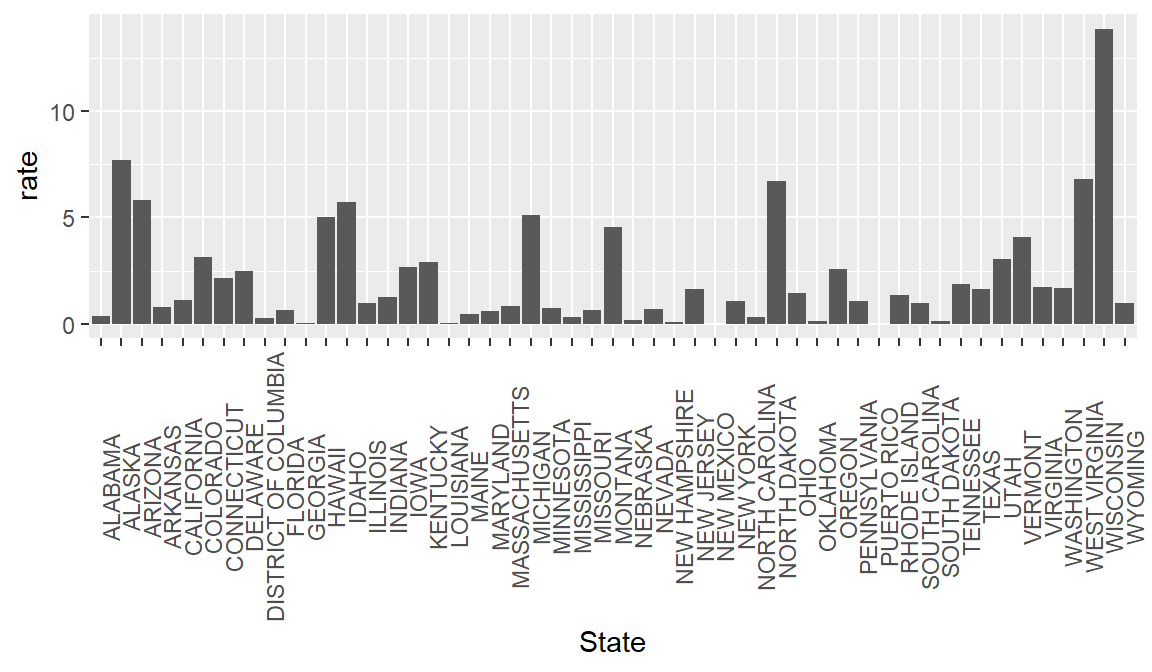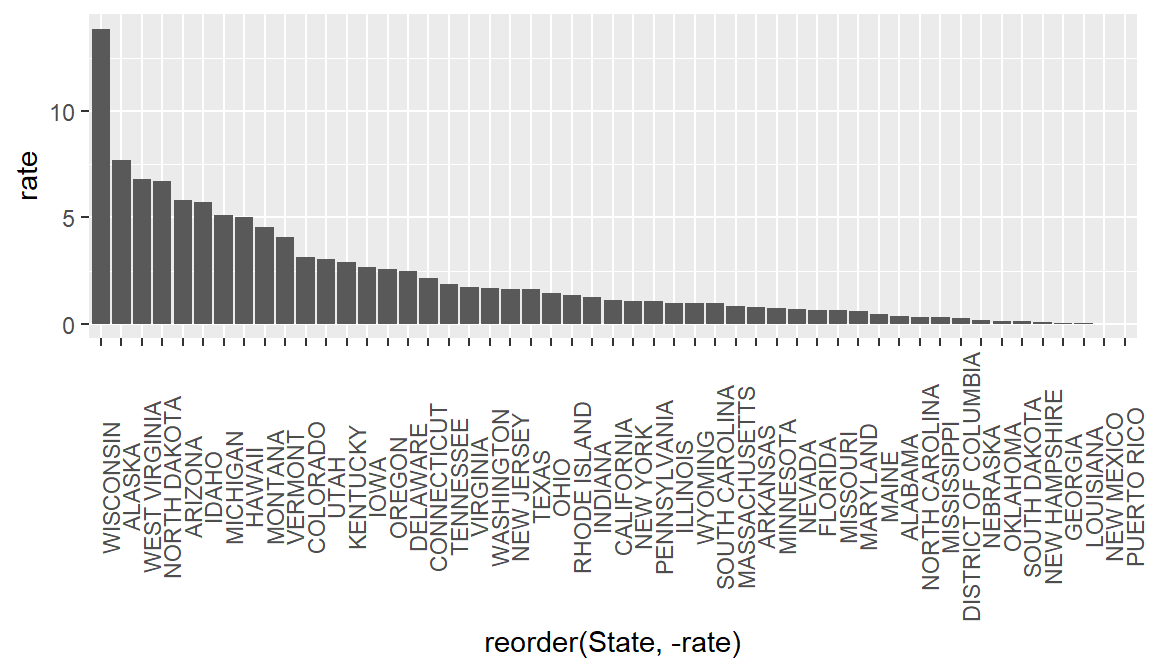## 6.1 Long vs Wide formats

For data to be considered “tidy,” it should be in what is called “long” format. Each column is a variable, each row is an observation, and each cell is a value. Our state population data is in “wide” format, which is often preferable for human-readability, but is less ideal for machine-readability.

We will use the package `tidyr` and the function `pivot_longer` to convert our population data to a long format, thus making it easier to join with our measles data.

Each column in our population dataset represents a state. To make it tidy we are going to reduce those to one column called State with the state names as the values of the column. We will then need to create a new column for population containing the current cell values. To remember that the population data is provided in 1000s of persons, we will call this new column pop1000.

`pivot_longer()` takes four principal arguments:

1. the data
2. cols are the names of the columns we use to fill the new values variable (or to drop).
3. the names_to column variable we wish to create from the cols provided.
4. the values_to column variable we wish to create and fill with values associated with the cols provided.
``````library(tidyr)
hist_pop_long <- hist_pop_by_state %>%
names_to = "State",
values_to = "pop1000")``````
``View(hist_pop_long)``

Now our two datasets have similar structures, a column of state names, a column of years, and a column of values. Let’s join these two datasets by the state and year columns. Note that if both sets have the same column names, you do not need to specify anything in the by argument. We use a left join here which preserves all the rows in our measles dataset and adds the matching rows from the population dataset.

``````joined_df <-
left_join(yearly_count_state, hist_pop_long, by=c("State" = "State", "Year" = "DATE" ))

joined_df``````
``````## # A tibble: 4,210 x 4
## # Groups:   Year 
##     Year State         TotalCount pop1000
##    <dbl> <chr>              <dbl>   <dbl>
##  1  1906 CALIFORNIA           224    1976
##  2  1906 CONNECTICUT           23    1033
##  3  1906 FLORIDA                4     628
##  4  1906 ILLINOIS             187    5309
##  5  1906 INDIANA               20    2663
##  6  1906 KENTUCKY               2    2234
##  7  1906 MAINE                 26     729
##  8  1906 MASSACHUSETTS        282    3107
##  9  1906 MICHIGAN             320    2626
## 10  1906 MISSOURI             274    3223
## # ... with 4,200 more rows``````

Now we can use our old friend `mutate()` to add a rate column calculated from the count and pop1000 columns.

``````#Add column for rate (per 1000) of measles
rate_by_year <- mutate(joined_df, rate = TotalCount / pop1000)
rate_by_year``````
``````## # A tibble: 4,210 x 5
## # Groups:   Year 
##     Year State         TotalCount pop1000     rate
##    <dbl> <chr>              <dbl>   <dbl>    <dbl>
##  1  1906 CALIFORNIA           224    1976 0.113
##  2  1906 CONNECTICUT           23    1033 0.0223
##  3  1906 FLORIDA                4     628 0.00637
##  4  1906 ILLINOIS             187    5309 0.0352
##  5  1906 INDIANA               20    2663 0.00751
##  6  1906 KENTUCKY               2    2234 0.000895
##  7  1906 MAINE                 26     729 0.0357
##  8  1906 MASSACHUSETTS        282    3107 0.0908
##  9  1906 MICHIGAN             320    2626 0.122
## 10  1906 MISSOURI             274    3223 0.0850
## # ... with 4,200 more rows``````

Now, let’s plot that data

``````rate_1963 <-
rate_by_year %>%
filter(Year == 1963) %>%
ggplot(aes(x = State, y = rate)) +
geom_bar(stat = "identity") +
theme(axis.text.x = element_text(angle = 90))

rate_1963``````
``## Warning: Removed 1 rows containing missing values (position_stack).``It can be helpful to look at bar graphs sorted

``````rate_1963 <-
rate_by_year %>%
filter(Year == 1963) %>%
ggplot(aes(x = reorder(State, -rate), y = rate)) +
geom_bar(stat = "identity") +
theme(axis.text.x = element_text(angle = 90))

rate_1963``````
``## Warning: Removed 1 rows containing missing values (position_stack).``